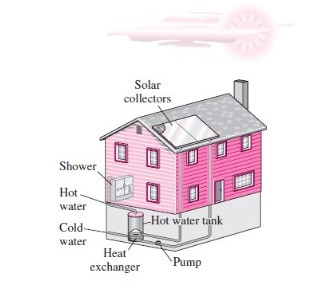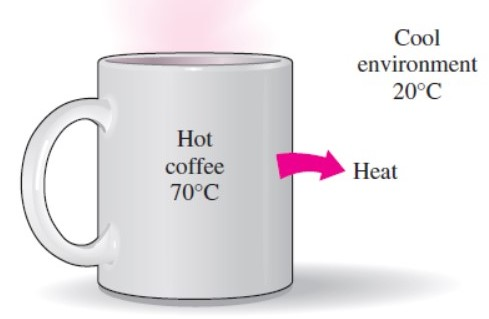USE PROMO CODE "DEMO100" TO AVAIL FREE DEMO CLASSThermodynamics

Thermodynamics can be defined as the science of energy. Energy can be defined as something which changes the physical property or chemical property of an object.

The word thermodynamics has been derived from Greek which means “heat power, which is the most descriptive of the earlier efforts to convert heat into power. Thermodynamics now is interpreted to include all aspects of energy and energy transformations, including power generation, refrigeration, and relationships among properties of matter.The design of many engineering system involves thermodynamics as shown in this figure.

Thermodynamics is defined by different laws which are known as the laws of the thermodynamics. There are three laws.

Laws of the thermodynamics:

1st Law of the thermodynamics: it simply states that during an interaction, energy can change from one form to another, but the total amount t of energy remains constant. That is energy can never be created or destroyed.

This law is also known as the conservation of energy principle. For example: a rock falling from a height is simply the conversion of the gravitational potential energy into kinetic energy.

2nd Law of thermodynamics: It simply states that energy has quality as well as quantity and the actual process occur in the direction of decreasing quality of energy. For example, a cup of hot coffee left on the table eventually cools but a cold coffee left on the same table never heats up. The high temperature energy of the coffee is degraded (transformed into less useful form of energy) as I it is transferred to the surrounding air.The heat flows in the direction from high temperature to low temperature

3rd Law of thermodynamics: The third law of thermodynamics states as follows, regarding the properties of closed systems in thermodynamic equilibrium:

The entropy of a system approaches a constant value as its temperature approaches absolute zero. At absolute zero the system must be in a state of least possible energy.

Zeroth law of the thermodynamics: It states that if two bodies are in thermal equilibrium with a third body, then they are also in thermal equilibrium with each other. Two systems can be said to be in thermal equilibrium with each other if they have the same temperature.

## Join As Tutor Or Student

Join our community that have more than 10,000 subscribers and learn new things everyday